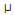# Electronics - Capacitors - Discussion

### Discussion :: Capacitors - General Questions (Q.No.6)

6.

When a 4.7F capacitor is connected to a 1 kHz source, what is its capacitive reactance?

 [A]. 4.7 ohms [B]. 29.5 ohms [C]. 34 ohms [D]. 213 ohms

Explanation:

No answer description available for this question.

 Seshendra said: (Nov 17, 2010) Capacitive reactance= 1/(2*pi*f*c).

 Avi said: (Aug 27, 2011) Any one explain this question.

 Ravi said: (Sep 21, 2011) Xc=1/Jwc =1/2*pi*f*c Keeping factor aside. As we interested mainly on reactance . So substituting in above relation.. We will get 1/2*3.12*1k*4.7micro =34 ohm

 Ravi said: (Sep 21, 2011) Xc=1/Jwc =1/2*pi*f*c Keeping factor aside. As we interested mainly on reactance . So substituting in above relation.. We will get 1/2*3.12*1k*4.7micro =34 ohm

 Muhammad Jamal said: (Feb 15, 2012) Xc=jwc as w=2*pi*f*c Keeping factor aside Xc=1/2*pi*f*c by putting the values we have =1/2*3.14*1000*4.7u =34 ohms

 Blessy Mathew said: (Aug 31, 2012) Xc=1/Jwc =1/2*pi*f*c

 Narendra said: (May 29, 2014) What calculated pi value?

 Kevin Ochieng said: (Nov 4, 2014) How is 34 coming?

 Prashanth Kotian said: (Jan 30, 2015) = 1/2x3.14x1000x4.7x10-6. = 1/2x3.14x1x4.7x10-3. = 1000/2x3.14x1x4.7. = 1000/29.516. = 34 ohms.

 Dan said: (Apr 27, 2016) @Prashanth Kotian. How 4.7 x 10-6 becomes 4.7 x 10-3?

 Mahesh said: (May 26, 2016) Xc = 1/(2 * 3.14 * f * c). = 1/(2 * 3.14 * 1000 * 0.0000047). = 33.86.

 Sk Sajahan said: (Nov 5, 2016) = 1/2 x 3.14 x 1000x4.7 x 10-6. = 1/2 x 3.14 x 1 x 4.7 1 0-3. = 1000/2 x 3.14 x 1 x 4.7. = 1000/29.516. = 34 ohms.